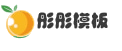# DedeCMS文章自动添加指定关键词链接的方法

2021-07-19 0 6,883
DedeCMS在该版本又对许多地方进行了改进。但是我一直期待的自动添加指定关键字链接功能却仍没有出现，而且目前关键词添加链接的次数还是不可以控制。于是我在DEDE论坛中仔细搜索，终于发现有一位朋友给出了自己的修改方案，此方案是目前5.3版中较好解决关键词链接问题方法

 `01` `foreach(\$kws as \$k) `
 `02` `{ `
 `03` `\$k = trim(\$k); `
 `04` `if``(\$k!=``""``) `
 `05` `{ `
 `06` `if``(\$i > \$maxkey) `
 `07` `{ `
 `08` `break``; `
 `09` `} `
 `10` `\$myrow = \$this->dsql->GetOne(``"select * from dede_keywords where keyword='\$k' And rpurl<>'' "``); `
 `11` `if``(is_array(\$myrow)) `
 `12` `{ `
 `13` `\$karr[] = \$k; `
 `14` `\$kaarr[] = ``"\$k"``; `
 `15` `} `
 `16` `\$i++; `
 `17` `}`

 `01` `\$dsql = new DedeSql(``false``); `
 `02`
 `03` `\$query=``"select * from dede_keywords where rpurl<>'' "``; `
 `04` `\$dsql->SetQuery(\$query); `
 `05` `\$dsql->Execute(); `
 `06` `while``(\$row = \$dsql->GetArray()) `
 `07` `{ `
 `08` `\$key = trim(\$row[``'keyword'``]); `
 `09` `\$rul=trim(\$row[``'rpurl'``]); `
 `10` `if``(\$rul!=``""``) `
 `11` `if``(!in_array(\$key, \$kw)) `
 `12` `if``(!in_array(\$kw,\$key)) `
 `13` `if``(substr_count(\$body,\$key)<5) `
 `14` `{ `
 `15` `\$karr[] = \$key; `
 `16` `\$kaarr[] = ``"\$key"``; `
 `17` `} `
 `18`
 `19` `}`• 模板团承诺演示效果图均和网站95%一样，并且我们的模板和数据都没有留后门，请放心使用。查看详情
• 因为模板具有可复制性和可传播性，模板一旦发货，非质量问题，不支持退换；所以务必请您一定看清楚后再购买。查看详情

### 相关文章3个月前 2,7843个月前 2,8693个月前 3,7073个月前 3,461

• 0 +

访问总数

• 0 +

会员总数

• 0 +

资源总数

• 0 +

今日发布

• 0 +

本周发布

• 0 +

运行天数